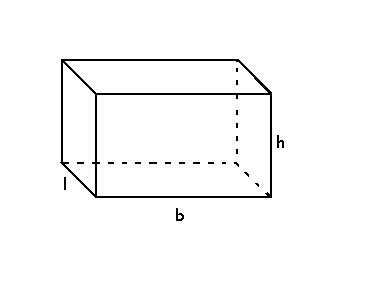# Surface Area of a Rectangular Prism Formula

A polyhedron with two polygonal bases parallel to each other is a prism.A Prism that has 2 parallel rectangular bases and 4 rectangular faces is a Rectangular Prism. The surface area of the rectangular prism is the sum of the area of lateral faces and rectangular bases. The unit of measurement for the surface area of rectangular prism is done in square units.

Given below is an illustration of rectangular prism:The Surface Area of a Rectangular Prism Formula is,

$$\begin{array}{l}\large Surface\;Area\;of\;a\;Rectangular\;Prism=2(bl+lh+hb)\end{array}$$

Where,
b – base length of the rectangular prism.
l – base width of the rectangular prism.
h – height of the rectangular prism.

### Solved Examples

Question 1: Find the surface area of a rectangular prism with base 6 cm, h = 12 cm and side 5 cm.

Solution:

Given,
b = 6 cm
l = 5 cm
h = 12 cm

Surface area of a rectangular prism

$$\begin{array}{l}=2(bl+lh+hb)\end{array}$$
$$\begin{array}{l}=2(6\times 12+12\times 5+5\times 6)\end{array}$$
$$\begin{array}{l}=2(72+60+30)\end{array}$$
$$\begin{array}{l}=324\;cm^{2}\end{array}$$